九路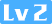960 0 0

### 深度优先遍历(DFS)

#### 前序遍历

``````class BST {
constructor() {
this.root = null // 根节点
}
...

preorder(fn) { // 前序遍历
const _helper = node => {
if (!node) {
return
}
fn(node.val) // 先访问根节点
_helper(node.left) // 再访问左节点
_helper(node.right) // 最后访问右节点
}
_helper(root)
}
} ``````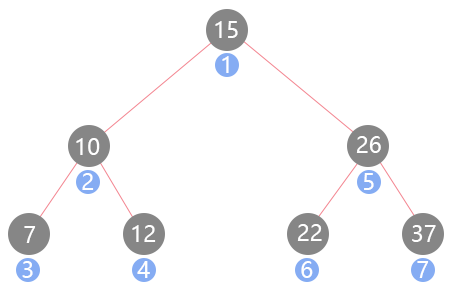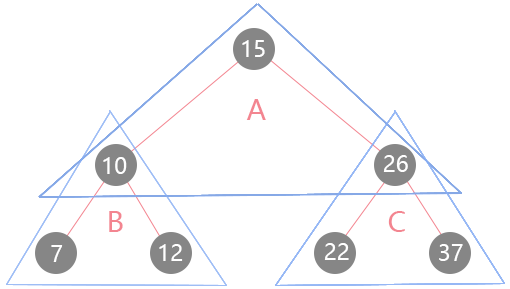#### 前序遍历应用 - 108-将有序数组转换为二叉搜索树

``````将一个按照升序排列的有序数组，转换为一棵高度平衡二叉搜索树。

0
/ \
-3   9
/   /
-10  5 ``````

``````function TreeNode(val) { // 树节点类
this.val = val;
this.left = this.right = null;
}

var sortedArrayToBST = function (nums) {
const _helper = (arr, l, r) => { // l为左边界、r为右边界
if (l > r) { // 递归终止条件
return null
}
const mid = l + (r - l) / 2 | 0 // 取数组中间值，并向下取整
const node = new TreeNode(arr[mid]) // 前序遍历，实例化为树节点
node.left = _helper(arr, l, mid - 1) // 将分割的左侧构建为二叉搜索树
node.right = _helper(arr, mid + 1, r) // 将分割的右侧构建为二叉搜索树
return node // 将构建好的树返回
}
return _helper(nums, 0, nums.length - 1) // 注意前闭后闭的区间方式
}; ``````

#### 中序遍历

``````class BST {
constructor() {
this.root = null // 根节点
}
...

inorder(fn) { // 中序遍历
const _helper = node => {
if (!node) {
return
}
_helper(node.left) // 先访问左孩子
fn(node.val) // 再访问根节点
_helper(node.right) // 最后访问右孩子
}
_helper(root)
}
} ``````

#### 中序遍历应用 - 530-二叉搜索树的最小绝对差

``给你一棵所有节点为非负值的二叉搜索树，请你计算树中任意两节点的差的绝对值的最小值。 ``

``````var getMinimumDifference = function (root) {
let prev = null // 保存之前访问的节点
let minimum = Infinity // 取最大值
const _helper = node => {
if (!node) {
return
}
_helper(node.left)
if (prev !== null) { // 第一次访问没有prev节点
minimum = Math.min(minimum, node.val - prev.val) // 始终保存最小的值
}
prev = node // 将本次循环的节点缓存为上一个节点
_helper(node.right)
}
_helper(root)
return minimum // 返回最小差
}; ``````

#### 后序遍历

``````class BST {
constructor() {
this.root = null // 根节点
}
...

postorder(fn) { // 后序遍历
const _helper = node => {
if (!node) {
return
}
_helper(node.left) // 先访问左孩子
_helper(node.right) // 然后访问右孩子
fn(node.val) // 最后访问根节点
}
_helper(root)
}
} ``````

#### 后序遍历应用 - 563-二叉树的坡度

``````给定一个二叉树，计算整个树的坡度。

``````var findTilt = function (root) {
let tilt = 0
const _helper = (node) => {
if (!node) {
return 0 // 叶子节点的坡度为0
}
const l = _helper(node.left) // 先求出左子树的和
const r = _helper(node.right) // 再求出右子树的和
tilt += Math.abs(l - r) // 当前节点的坡度等于左右子树和的绝对差
return l + r + node.val // 返回子树和
}
_helper(root)
return tilt
}; ``````

### 深度优先遍历(DFS)应用进阶

#### 反常规中序遍历 - 538-把二叉搜索树转换为累加树

``给定一个二叉搜索树，把它转换成为累加树，使得每个节点的值是原来的节点值加上所有大于它的节点值之和。 ``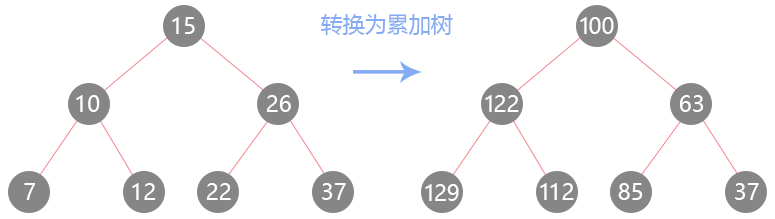``````var convertBST = function (root) {
let sum = 0
const _helper = (node) => {
if (!node) {
return null
}
_helper(node.right) // 先遍历右子树
node.val += sum // 右子树到底后逐个节点进行值的累加与覆盖
sum = node.val // 记录的就是上个被覆盖节点的值
_helper(node.left) // 最后遍历左子树
}
_helper(root)
return root // 返回新的累加树
}; ``````

#### 前序加后序遍历 - 257-二叉树的所有路径

``给定一个二叉树，返回所有从根节点到叶子节点的路径。 ``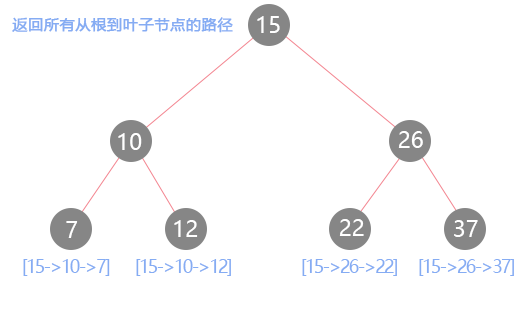``````var binaryTreePaths = function (root) {
const ret = []
const _helper = (node, path) => {
if (!node) {
return
}
path.push(node.val) // 前序遍历访问，记录路径的每一步
_helper(node.left, path)
_helper(node.right, path)
if (!node.left && !node.right) { // 到达了叶子节点
ret.push(path.join('->')) // 将路径转换为字符串
}
path.pop() // 后序遍历访问，将访问过的路径节点弹出
}
_helper(root, [])
return ret
}; ``````

### 广度优先遍历(BFS)

#### 树的BFS实现

``````class BST {
constructor() {
this.root = null // 根节点
}
...

levelOrder(fn) {
if (!this.root) {
return
}
const queue = [this.root] // 首先入队根节点
while (queue.length > 0) {
const node = queue.shift() // 出队队首节点
fn(node.val) // 访问出队节点值
if (node.left) { // 入队左孩子
queue.push(node.left)
}
if (node.right) { // 入队右孩子
queue.push(node.right)
}
}
}
} ``````

#### 广度优先遍历应用 - 637-二叉树的层平均值

``````var averageOfLevels = function (root) {
if (root === null) {
return []
}
const queue = [root]
const ret = [] // 最终返回的结果
while (queue.length > 0) {
let len = queue.length
let sum = 0 // 记录每一层的总和
for (let i = 0; i < len; i++) { // 遍历每一层
const node = queue.shift()
sum += node.val
if (node.left) {
queue.push(node.left)
}
if (node.right) {
queue.push(node.right)
}
}
ret.push(sum / len) // 求出该层的平均
}
return ret
}; ``````

### 相关推荐

JavaScript 中的二叉树以及二叉搜索树的实现及应用

Task4 建模与调参 4.1 学习目标 学习机器学习模型的建模过程与调参流程 完成相应学习打卡任务 4.2 内容介绍 逻辑回归模型： 理解逻辑回归模型； 逻辑回归模型的应用； 逻辑回归的优缺点； 树模型： 理解树模型； 树模型的应用； 树模型的优缺点； 集成模型 基于bagging思想的集成
js实现二叉树、二叉查找树

DS博客作业05
#1.本周学习总结 ##1.思维导图 ![](https://oscimg.oschina.net/oscnet/7226aa2f8d9507a45bebcc4789d5f40ccee.png) ##2.谈谈你对树结构的认识及学习体会 相对于之前的栈和队列,树的学习难度明显. 相较于之前的线性结构, 树更多的需要应用递归思维. 循环结构用多了之后,
JAVA递归实现线索化二叉树
JAVA递归实现线索化二叉树 ============== ### 基础理论 首先，二叉树递归遍历分为先序遍历、中序遍历和后序遍历。 先序遍历为：根节点+左子树+右子树 中序遍历为：左子树+根节点+右子树 后序遍历为：左子树+右子树+根节点 （只要记住根节点在哪里就是什么遍历，且都是先左再右） ### 线索化 现在有这么一棵二叉树，它的数据结
Java数据结构和算法（十五）——无权无向图

444，二叉树的序列化与反序列化
![](https://oscimg.oschina.net/oscnet/2af309d8-a9c9-4452-a07b-615ec9c55b2e.png) It's easy to find if you know what you're looking for. 如果你知道自己想追求什么，就很容易成功。 **问题描述**
20175317 《Java程序设计》第四周学习总结
20175317 《Java程序设计》第四周学习总结 ========================== 教材学习内容总结 -------- 第四周我学习了教材第五章的内容，了解了子类与继承的知识，学到了以下内容： 明白了什么是子类与父类、类的树形结构。 子类和父类在同一包中、不在同一包中时的继承性。 子类与对象，子类对象的特点。 成员变量的隐藏与方
20175317 《Java程序设计》第四周学习总结
20175317 《Java程序设计》第四周学习总结 ========================== 教材学习内容总结 -------- 第四周我学习了教材第五章的内容，了解了子类与继承的知识，学到了以下内容： 明白了什么是子类与父类、类的树形结构。 子类和父类在同一包中、不在同一包中时的继承性。 子类与对象，子类对象的特点。 成员变量的隐藏与方
Javascript解析机制 执行机制
HTML5学堂：在学习JavaScript过程中，我们需要了解事件的机制是怎么执行的？本文将会提到JavaScript事件机制的解析，希望对大家有帮助！ javascript解析的过程主要分为两个阶段，分别是编译与执行阶段。 在编译期，javascript解释器将完成对javascript代码的预处理，即将javascript代码转换为字节码。 在执行
LeetCode（94）：二叉树的中序遍历
**Medium！** **题目描述：** 给定一个二叉树，返回它的_中序_ 遍历。 示例: 输入: [1,null,2,3] 1 \ 2 / 3 输出: [1,3,2] 进阶: 递归算法很简单，你可以通过迭代算法完成吗？ **解题思路：**
React的虚拟DOM

HelloWorld开发者社区 - 开发者专属的技术社区